# 50 Challenging Calculus Problems (Fully Solved)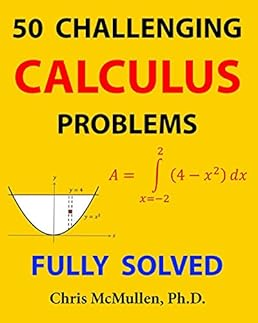Price: \$9.99
(as of Apr 13,2022 13:34:58 UTC – Details)

From the Publisher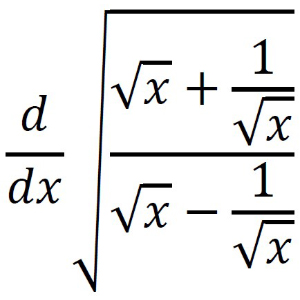### 50 Challenging Calculus Problems (Fully Solved) by Chris McMullen, Ph.D.

Improve your calculus fluency by solving a variety of challenging calculus problems.Practice your calculus skills, including derivatives, integration techniques, limits, and more.Being confronted with many different types of problems out of the usual context helps to develop problem-solving skills.The full solution is included for every problem.The level of difficulty varies. After attempting a harder problem, reading the full solution will be instructive.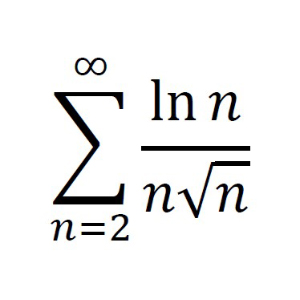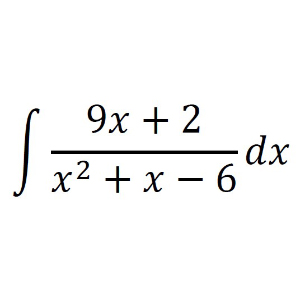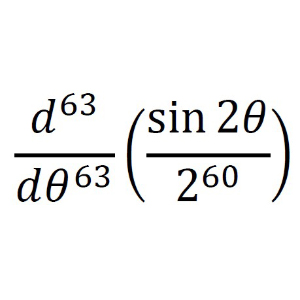Does this series converge or diverge?

Does this function increase or decrease as the series progresses? Does the initial value matter? Is there a convergence test for this series?

Find the antiderivative.

Which technique of integration would help for this indefinite integral?

Find the derivative.

This problem should be relatively straightforward.

Number of Pages
257 125 234 151 112 173

Full solutions to all problems. Answers + some hints, solutions, and tips. Answers + some hints, solutions, and tips. Full solutions to every problem. Full solutions to every problem. Full solutions to every problem.

ISBN
1941691927 1941691382 1941691323 1941691242 1941691269 1941691374

Description
Challenge yourself with a variety of algebra problems that will make you think. The solutions are instructive. Comprehensive coverage of trig identities. Master logarithms and exponentials with in-depth coverage, including identities, the change of base formula, graphs, hyperbolic functions, applications, and even complex numbers. Build fluency by practicing essential calculus skills. Not just for the Einsteins of the world, anyone fluent with calculus can challenge their skills. Multivariable calculus and vector calculus.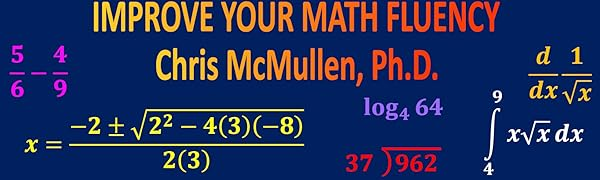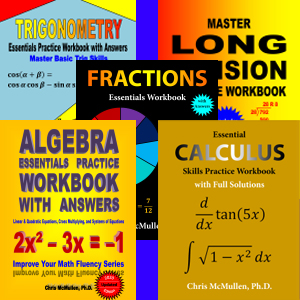Chris McMullen, Ph.D.

Learn math from a university instructor with over 20 years of teaching experience.

Build Essential Math Skills

Practice solving problems that build essential math skills.Check your answers at the back of the book to ensure that practice makes perfect. Develop Confidence

When you check your answer at the back of the book and discover that your solution is correct, this helps to build confidence.Fully solved examples and concise explanations help get you on the right path.

Find the subject that’s right for you. Arithmetic Prealgebra Algebra Geometry Trigonometry Logarithms Calculus Vector Calculus

Publisher ‏ : ‎ Zishka Publishing (September 2, 2018)
Language ‏ : ‎ English
Paperback ‏ : ‎ 112 pages
ISBN-10 ‏ : ‎ 1941691269
ISBN-13 ‏ : ‎ 978-1941691267
Item Weight ‏ : ‎ 8.5 ounces
Dimensions ‏ : ‎ 8 x 0.26 x 10 inches